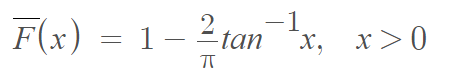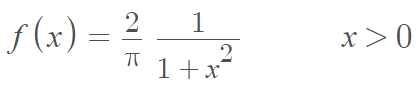# Half-Cauchy Distribution

Share on

The half-Cauchy distribution is a continuous probability distribution derived from folding the standard Cauchy distribution on the origin (i.e., on the peak). This creates a version of the Cauchy distribution with only positive values. In other words, the half-Cauchy is a Cauchy distribution with a domain restricted to only positive values. In some rare cases, the left half of the Cauchy might be the distribution of interest, in which case this is usually specified. If unspecified, assume the right-hand half (positive values).

The mean and variance are not defined.

## Definition of the Half-Cauchy Distribution

A continuous random variable X has a half-Cauchy distribution if its survival function isFrom this definition, we can derive the probability density functionThe half-Cauchy distribution is heavy tailed and self-decomposable  (a self-decomposable random variable has the same distribution as the sum of a scaled down version of itself and an independent residual random variable) . The half-Cauchy is also infinity divisible .

## References

 Jacob, E. & Jayakumar, K. On Half-Cauchy Distribution and Process. International Journal of Statistika and Mathematika, ISSN: 2277- 2790 E-ISSN: 2249-8605, Volume 3, Issue 2, 2012 pp 77-81
 A. Diedhiou. On the self-decomposability of the half-Cauchy distribution. J. MAth. Anal. Appl. 220(1), 42-64. 1998.
 Carr, P. et. al. (2007). Self-Decomposability and Option Pricing. Mathematical Finance, Vol. 17, No. 1 (January 2007), 31–57
 L. Bondesson, on the infinite divisibility of the half-Cauchy and other decreasing densities and probability functions on the nonnegative line. Scand. Actua. J. 1987(3), 225-247 (1987).

CITE THIS AS:
Stephanie Glen. "Half-Cauchy Distribution" From StatisticsHowTo.com: Elementary Statistics for the rest of us! https://www.statisticshowto.com/half-cauchy-distribution/
---------------------------------------------------------------------------Need help with a homework or test question? With Chegg Study, you can get step-by-step solutions to your questions from an expert in the field. Your first 30 minutes with a Chegg tutor is free!